# 在 EA 交易代码中实现指标的计算

20 八月 2018, 09:08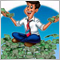0
8 094

### 1. 代码迁移的原则

```//+------------------------------------------------------------------+
//|                                                        Blanc.mq5 |
//|                                 http://www.mql5.com/ru/users/dng |
//+------------------------------------------------------------------+
#property version   "1.00"
#property indicator_chart_window
#property indicator_buffers 1
#property indicator_plots   1
//--- 绘图缓冲区
#property indicator_label1  "Buffer"
#property indicator_type1   DRAW_LINE
#property indicator_color1  clrRed
#property indicator_style1  STYLE_SOLID
#property indicator_width1  1
//--- 指标缓冲区
double         BufferBuffer[];
//+------------------------------------------------------------------+
//| 自定义指标初始化函数                                                 |
//+------------------------------------------------------------------+
int OnInit()
{
//--- 指标缓冲区映射
SetIndexBuffer(0,BufferBuffer,INDICATOR_DATA);

//---
return(INIT_SUCCEEDED);
}
//+------------------------------------------------------------------+
//| 自定义指标迭代函数                                                  |
//+------------------------------------------------------------------+
int OnCalculate(const int rates_total,
const int prev_calculated,
const datetime &time[],
const double &open[],
const double &high[],
const double &low[],
const double &close[],
const long &tick_volume[],
const long &volume[],
{
//---

//--- 返回 prev_calculated 的值用于下一次调用
return(rates_total);
}
//+------------------------------------------------------------------+
```

### 2. 创建指标计算的类

1. 准备指标缓冲区的操作，为此，创建 CArrayBuffer 类，带有用于数据存储和方便访问的方法。随后，根据指标中缓冲区的数量来创建这样的类的数组。
2. 把来自 OnCalculate 函数中指标的计算部分迁移到我们类的 Calculate 函数中。
3. 指标可以从 OnCalculate 函数参数中访问时间序列 (和 EA 的函数不同),所以，我们要在 LoadHistory 函数中处理所需时间序列的下载。
4. 为了统一访问重新计算的指标数据，让我们在 CIndicator 类中使用所有必须的参数来创建 CopyBuffer 函数。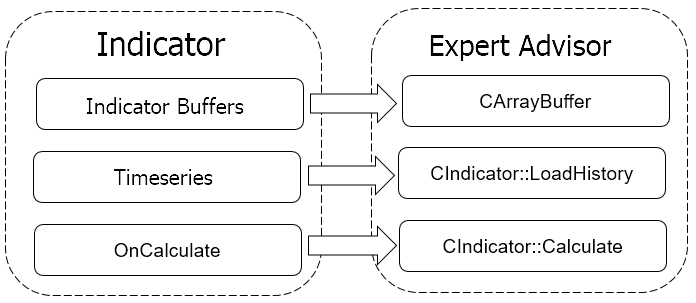#### 2.1. 创建指标缓冲区

```class CArrayBuffer   :  public CArrayDouble
{
public:
CArrayBuffer(void);
~CArrayBuffer(void);
//---
int               CopyBuffer(const int start, const int count, double &double_array[]);
int               Initilize(void);
virtual bool      Shift(const int shift);
};
```

#### 2.2. 用于将来指标的父类

```class CIndicator
{
private:
//---
public:
CIndicator(void);
~CIndicator(void);
virtual bool         Create(const string symbol=NULL, const ENUM_TIMEFRAMES timeframe=PERIOD_CURRENT, const ENUM_APPLIED_PRICE price=PRICE_CLOSE);
//--- 设置指标的主要配置
virtual bool         SetBufferSize(const int bars);
//--- 取得指标数据
virtual int          CopyBuffer(const uint buffer_num,const uint start, const uint count, double &double_array[]);
virtual double       GetData(const uint buffer_num,const uint shift);

protected:
double               m_source_data[];
CArrayBuffer         ar_IndBuffers[];
int                  m_buffers;
int                  m_history_len;
int                  m_data_len;
//---
string               m_Symbol;
ENUM_TIMEFRAMES      m_Timeframe;
ENUM_APPLIED_PRICE   m_Price;
//--- 设置指标的主要配置
virtual bool         SetHistoryLen(const int bars=-1);
//---
virtual bool         Calculate()                         {  return true;   }
};
```

• 构造函数,
• 析构函数,
• 类初始化方法,
• 用于指定指标缓冲区大小的方法
• 两个用于访问指标数据的方法: 第一个用于批量数据的下载，而第二个是用于访问特定的元素。

• 用于计算的初始数据数组 (m_source_data);
• 指标缓冲区的数组 (ar_IndBuffers);
• 用于保存指标缓冲区数量的变量 (m_buffers), 所需初始数据历史的深度 (m_history_len) 以及所需指标值历史的深度 (m_data_len);
• 使用的交易品种 (m_Symbol) 和时段 (m_Timeframe);
• 用于指标计算的价格类型 (m_Price);
• 用于配置的方法: 初始化数据深度 (SetHistoryLen); 时段数据历史的下载 (LoadHistory); 指标的重新计算 (Calculate).

```CIndicator::CIndicator()   :  m_buffers(0),
m_Symbol(_Symbol),
m_Timeframe(PERIOD_CURRENT),
m_Price(PRICE_CLOSE),
{
m_data_len=m_history_len  =  Bars(m_Symbol,m_Timeframe)-1;
ArrayFree(ar_IndBuffers);
ArrayFree(m_source_data);
}
```

```bool CIndicator::Create(const string symbol=NULL,const ENUM_TIMEFRAMES timeframe=0,const ENUM_APPLIED_PRICE price=1)
{
m_Symbol=(symbol==NULL ? _Symbol : symbol);
if(!SymbolInfoInteger(m_Symbol,SYMBOL_SELECT))
if(!SymbolSelect(m_Symbol,true))
return false;
//---
m_Timeframe=timeframe;
m_Price=price;
//---
return true;
}
```

```bool CIndicator::SetBufferSize(const int bars)
{
if(bars>0)
m_data_len  =  bars;
else
m_data_len  =  Bars(m_Symbol,m_Timeframe);
//---
if(m_data_len<=0)
{
for(int i=0;i<m_buffers;i++)
ar_IndBuffers[i].Shutdown();
return false;
}
//---
if(m_history_len<m_data_len)
if(!SetHistoryLen(m_data_len))
return false;
//---
for(int i=0;i<m_buffers;i++)
{
ar_IndBuffers[i].Shutdown();
if(!ar_IndBuffers[i].Resize(m_data_len))
return false;
}
//---
return true;
}
```

```bool CIndicator::LoadHistory(void)
{
datetime cur_date=(datetime)SeriesInfoInteger(m_Symbol,m_Timeframe,SERIES_LASTBAR_DATE);
return true;
//---
MqlRates rates[];
int total=0,i;
switch(m_Price)
{
case PRICE_CLOSE:
total=CopyClose(m_Symbol,m_Timeframe,1,m_history_len,m_source_data);
break;
case PRICE_OPEN:
total=CopyOpen(m_Symbol,m_Timeframe,1,m_history_len,m_source_data);
case PRICE_HIGH:
total=CopyHigh(m_Symbol,m_Timeframe,1,m_history_len,m_source_data);
case PRICE_LOW:
total=CopyLow(m_Symbol,m_Timeframe,1,m_history_len,m_source_data);
case PRICE_MEDIAN:
total=CopyRates(m_Symbol,m_Timeframe,1,m_history_len,rates);
if(total!=ArraySize(m_source_data))
total=ArrayResize(m_source_data,total);
for(i=0;i<total;i++)
m_source_data[i]=(rates[i].high+rates[i].low)/2;
break;
case PRICE_TYPICAL:
total=CopyRates(m_Symbol,m_Timeframe,1,m_history_len,rates);
if(total!=ArraySize(m_source_data))
total=ArrayResize(m_source_data,total);
for(i=0;i<total;i++)
m_source_data[i]=(rates[i].high+rates[i].low+rates[i].close)/3;
break;
case PRICE_WEIGHTED:
total=CopyRates(m_Symbol,m_Timeframe,1,m_history_len,rates);
if(total!=ArraySize(m_source_data))
total=ArrayResize(m_source_data,total);
for(i=0;i<total;i++)
m_source_data[i]=(rates[i].high+rates[i].low+2*rates[i].close)/4;
break;
}
//---
if(total<=0)
return false;
//---
return (total>0);
}
```

```int CIndicator::CopyBuffer(const uint buffer_num,const uint start,const uint count,double &double_array[])
{
if(!Calculate())
return -1;
//---
if((int)buffer_num>=m_buffers)
{
ArrayFree(double_array);
return -1;
}
//---
return ar_IndBuffers[buffer_num].CopyBuffer(start,count,double_array);
}
```

#### 2.3. 移动平均的指标类

```class CMA : public CIndicator
{
private:
int               m_Period;
int               m_Shift;
ENUM_MA_METHOD    m_Method;
datetime          m_last_calculate;

public:
CMA();
~CMA();
bool              Create(const string symbol, const ENUM_TIMEFRAMES timeframe, const int ma_period, const int ma_shift, const ENUM_MA_METHOD ma_method, const ENUM_APPLIED_PRICE price=PRICE_CLOSE);
virtual bool      SetBufferSize(const int bars);

protected:
virtual bool      Calculate();
virtual double    CalculateSMA(const int shift);
virtual double    CalculateEMA(const int shift);
virtual double    CalculateLWMA(const int shift);
virtual double    CalculateSMMA(const int shift);
};
```

```CMA::CMA()  :  m_Period(25),
m_Shift(0),
m_Method(MODE_SMA)
{
m_buffers=1;
ArrayResize(ar_IndBuffers,1);
}
```

```bool CMA::Create(const string symbol,const ENUM_TIMEFRAMES timeframe,const int ma_period,const int ma_shift,const ENUM_MA_METHOD ma_method,const ENUM_APPLIED_PRICE price=1)
{
if(!CIndicator::Create(symbol,timeframe,price))
return false;
//---
if(ma_period<=0)
return false;
//---
m_Period=ma_period;
m_Shift=ma_shift;
m_Method=ma_method;
//---
if(!SetBufferSize(ma_period))
return false;
if(!SetHistoryLen(2*ma_period+(m_Shift>0 ? m_Shift : 0)))
return false;
//---
return true;
}
```

Calculate 函数会直接计算指标。当创建父类的时候，我们决定当开启新的烛形时会载入时间序列的历史数据，所以，我们将会以同样的频率来重新计算指标数据。为此，我们要在函数的开始检查是否有新的烛形开启，如果已经在当前柱上进行过计算，就以'true'的结果退出函数，

```bool CMA::Calculate(void)
{
datetime cur_date=(datetime)SeriesInfoInteger(m_Symbol,m_Timeframe,SERIES_LASTBAR_DATE);
if(m_last_calculate==cur_date && ArraySize(m_source_data)==m_history_len)
return true;
//---
return false;
//---
int shift=Bars(m_Symbol,m_Timeframe,m_last_calculate,cur_date)-1;
if(shift>m_data_len)
{
ar_IndBuffers.Initilize();
shift=m_data_len;
}
else
ar_IndBuffers.Shift(shift);
//---
for(int i=(m_data_len-shift);i<m_data_len;i++)
{
int data_total=ar_IndBuffers.Total();
switch(m_Method)
{
case MODE_SMA:
if(i>=data_total)
else
ar_IndBuffers.Update(i,CalculateSMA(i+m_Shift));
break;
case MODE_EMA:
if(i>=data_total)
else
ar_IndBuffers.Update(i,CalculateEMA(i+m_Shift));
break;
case MODE_SMMA:
if(i>=data_total)
else
ar_IndBuffers.Update(i,CalculateSMMA(i+m_Shift));
break;
case MODE_LWMA:
if(i>=data_total)
else
ar_IndBuffers.Update(i,CalculateLWMA(i+m_Shift));
break;
}
}
//---
m_last_calculate=cur_date;
m_data_len=ar_IndBuffers.Total();
//---
return true;
}
```

### 3. 把指标类加到 EA 交易中的例子

```input int                  MA_Period   =  25;
input int                  MA_Shift    =  0;
input ENUM_MA_METHOD       MA_Method   =  MODE_SMA;
input ENUM_APPLIED_PRICE   MA_Price    =  PRICE_CLOSE;
```

```CMA   *MA;
double c_data[];
```

```int OnInit()
{
//---
MA=new CMA;
if(CheckPointer(MA)==POINTER_INVALID)
return INIT_FAILED;
//---
if(!MA.Create(_Symbol,PERIOD_CURRENT,MA_Period,MA_Shift,MA_Method,MA_Price))
return INIT_FAILED;
MA.SetBufferSize(3);
//---
return(INIT_SUCCEEDED);
}
```

```void OnDeinit(const int reason)
{
//---
if(CheckPointer(MA)!=POINTER_INVALID)
delete MA;
}
```

```void OnTick()
{
//---
static datetime last_bar=0;
datetime cur_date=(datetime)SeriesInfoInteger(_Symbol,PERIOD_CURRENT,SERIES_LASTBAR_DATE);
if(last_bar==cur_date)
return;
last_bar=cur_date;
//---
if(!MA.CopyBuffer(MAIN_LINE,0,3,c_data))
return;

//---
//     在这里加上您用于处理信号和交易操作的代码
//---
return;
}
```

### 4. 使用迁移过的指标的"花费"

#### 4.1. 实验 1

• 通过终端中内建的指标函数 (iMA) ;
• 通过调用类似的自定义指标 (iCustom);
• 通过直接在 EA 内计算.

OnTick
99.14%

21.524 2.36%
包含 CopyClose 1.729  0.19%
iMA  2.231  0.24%
iCustom  0.748  0.08%
OnInit  241439  0.86%
接收 iCustom 句柄  235676  0.84%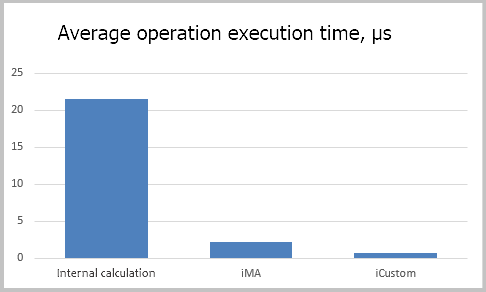#### 4.2. 实验 2

1. 一个标本空白 EA 不进行时间的计算。
2. 一个 EA 接收在指标类中计算数值的数据。
3. 一个 EA 接受来自 iMA 指标的数据。
4. 一个 EA 接受来自自定义指标的数据。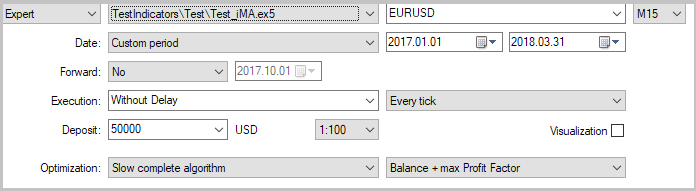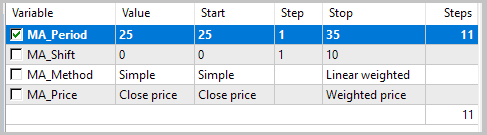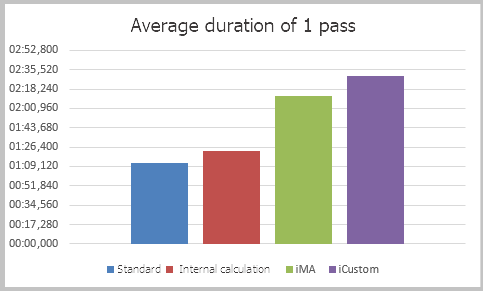#### 4.3. 实验 3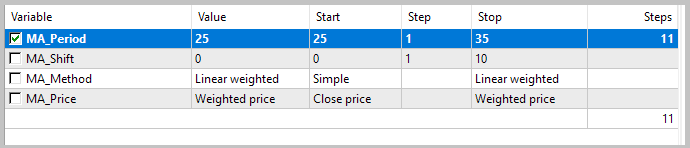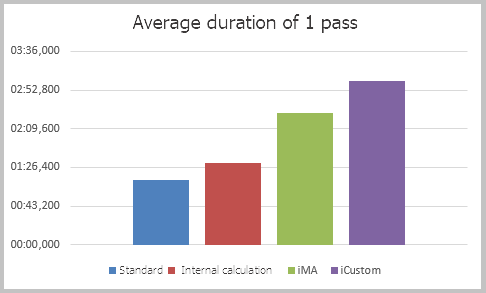### 本文中使用的程序

#
名称

1 Indicarot.mqh  类库  用于迁移指标的基类
2 MA.mqh  类库  用于在 EA 内计算 MA 指标的类
3 Test.mq5  EA  用于进行实验1的 EA
4 Test_Class.mq5  EA  在交易机器人内部进行指标计算的 EA (实验 2 和 3)
5 Test_iMA.mq5  EA  通过 iMA 来取得指标数据的 EA
6 Test_iCustom.mq5  EA  通过 iCustom 来取得指标数据的 EA

MQL5.zip (164.68 KB)

#### 该作者的其他文章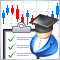如何创建订购交易机器人的需求规范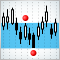10 种横盘交易策略的比较分析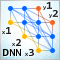深度神经网络 (第七部分)。 神经网络的融合: 堆叠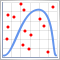蒙特卡洛方法在交易策略优化中的应用